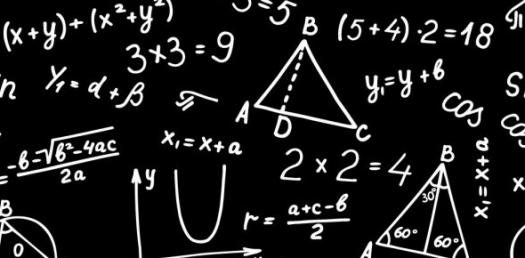Approved & Edited by ProProfs Editorial Team
At ProProfs Quizzes, our dedicated in-house team of experts takes pride in their work. With a sharp eye for detail, they meticulously review each quiz. This ensures that every quiz, taken by over 100 million users, meets our standards of accuracy, clarity, and engagement.
| Written by Robertmclellan03
R
Robertmclellan03
Community Contributor
Quizzes Created: 1 | Total Attempts: 1,261
Questions: 5 | Attempts: 1,261SettingsThis quiz will test you if you really know algebra

• 1.

### Subtract the following trinomes:(2y+3zx-4)-(5zx+3-6y)

• A.

8y-2zx+1

• B.

5y+4-4zx

• C.

-y

• D.

2zx-5+16y

A. 8y-2zx+1
Explanation
The given expression is a subtraction of two trinomials. To subtract them, we need to distribute the negative sign to the second trinomial and then combine like terms. By doing so, we get 2y + 3zx - 4 - 5zx - 3 + 6y. Combining like terms, we have 8y - 2zx + 1, which matches the given answer.

Rate this question:

• 2.

### Developpe the follweing equation:3(z-7+6y)

• A.

21-18z+7

• B.

-z+18y

• C.

3z-21+18y

• D.

3-7+z

• E.

-18y-z-7

C. 3z-21+18y
Explanation
The given equation is developed by distributing the 3 to each term inside the parentheses. This results in 3z - 21 + 18y, which is the correct answer.

Rate this question:

• 3.

### Multiply the following:(-2jrl)(-3yuh)(-4asd)

• A.

-24jrlyuhasd

• B.

24jrlyuhasd

• C.

-24jya

• D.

Jrlyuhasd

A. -24jrlyuhasd
Explanation
The given expression involves multiplying three terms: (-2jrl), (-3yuh), and (-4asd). When multiplying three negative numbers, the result is positive. Therefore, the correct answer is 24jrlyuhasd, without the negative sign.

Rate this question:

• 4.

### Developp the following, then simplify:(x+10)(x+2)

• A.

X2+5

• B.

X+20

• C.

2x+10-2

• D.

X2+12x+20

D. X2+12x+20
Explanation
The given expression is a product of two binomials. To simplify it, we can use the distributive property. Multiplying the first terms of each binomial gives x^2. Multiplying the outer terms gives 2x, and multiplying the inner terms gives 10x. Finally, multiplying the last terms gives 20. Combining like terms, we have x^2 + 12x + 20, which is the simplified form of the expression.

Rate this question:

• 5.

### Write a short, one paragraph essay on how to factorise polynomes with using simple trinome.

Related TopicsBack to top
×

Wait!
Here's an interesting quiz for you.